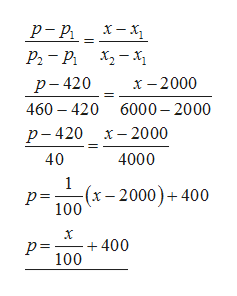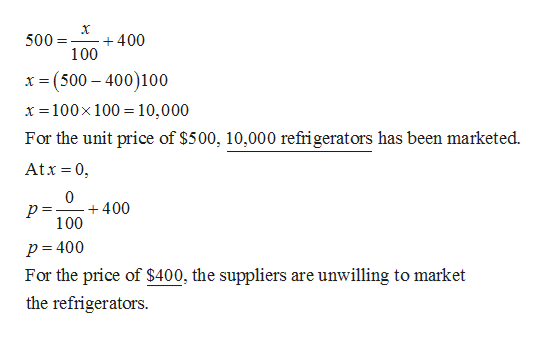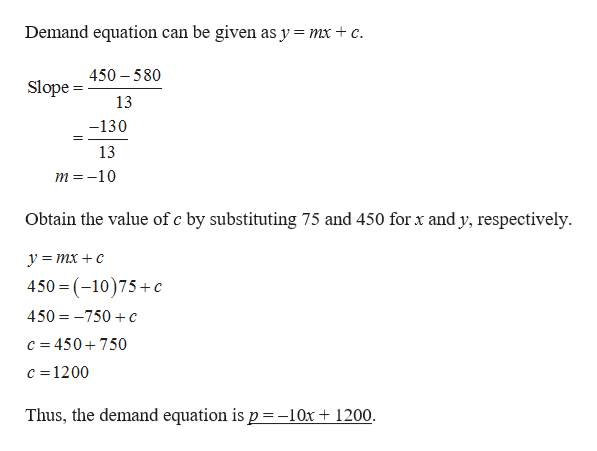# Producers will make 2000 refrigerators available when the unit price is \$420. At a unit price of \$460, 6000 refrigerators will be marketed. Find the equation relating the unit price p of a refrigerator to the quantity supplied x if the equation is known to be linear.p =  How many refrigerators will be marketed when the unit price is \$500?refrigeratorsAt what price will suppliers be unwilling to market refrigerators?   At a unit price of \$450, the quantity demanded of a certain commodity is 75 pounds. If the unit price increases to \$580, the quantity demanded decreases by 13 pounds. Find the demand equation (assuming it is linear) where p is the unit price and x is the quantity demanded for this commodity in pounds.p =  At what price are no consumers willing to buy this commodity?\$ According to the above model, how many pounds of this commodity would consumers take if it was free?pounds

Question
15 views

Producers will make 2000 refrigerators available when the unit price is \$420. At a unit price of \$460, 6000 refrigerators will be marketed. Find the equation relating the unit price p of a refrigerator to the quantity supplied x if the equation is known to be linear.
p =

How many refrigerators will be marketed when the unit price is \$500?
refrigerators

At what price will suppliers be unwilling to market refrigerators?

At a unit price of \$450, the quantity demanded of a certain commodity is 75 pounds. If the unit price increases to \$580, the quantity demanded decreases by 13 pounds. Find the demand equation (assuming it is linear) where p is the unit price and x is the quantity demanded for this commodity in pounds.
p =

At what price are no consumers willing to buy this commodity?
\$

According to the above model, how many pounds of this commodity would consumers take if it was free?
pounds

check_circle

Step 1

For x = 2000, p = \$420 and for x = 6000, p= \$460.

Equation is,help_outlineImage Transcriptioncloseх — х, р-р Р. — Р. х, —х х - 2000 р-420 460 420 6000-2000 р-420 х- 2000 40 4000 1 (х - 2000) + 400 р3 100 х +400 100 р- fullscreen
Step 2

When p = \$500,help_outlineImage Transcriptionclose+400 100 500= x (500 400)100 x 100x 100 10,000 For the unit price of \$500, 10,000 refrigerators has been marketed Atx 0 0 +400 100 p= 400 For the price of \$400, the suppliers are unwilling to market the refrigerators fullscreen
Step 3

Demand equation can be given as y = ...help_outlineImage TranscriptioncloseDemand equation can be given as y = mx + c 450 580 Slope 13 -130 13 m -10 Obtain the value of c by substituting 75 and 450 for x and y, respectively ymxc 450 (10)75+c 450 750c c 450750 c 1200 Thus, the demand equation is p =-10x 1200 fullscreen

### Want to see the full answer?

See Solution

#### Want to see this answer and more?

Solutions are written by subject experts who are available 24/7. Questions are typically answered within 1 hour.*

See Solution
*Response times may vary by subject and question.
Tagged in

### Equations and In-equations